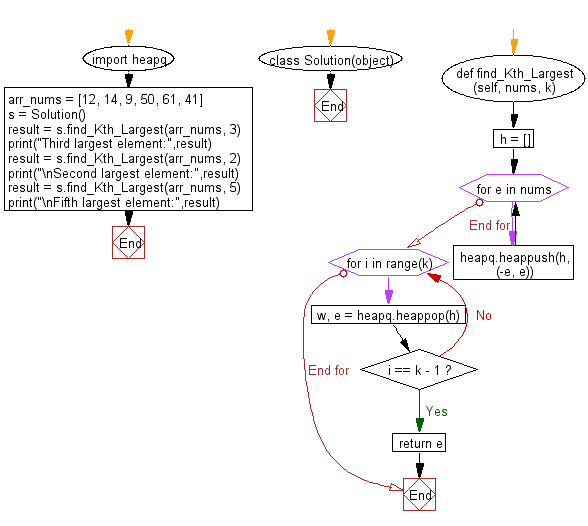﻿ Python heap queue algorithm: Find the kth largest element in an unsorted array using Heap queue algorithm - w3resource

# Python: Find the kth largest element in an unsorted array using Heap queue algorithm

## Python heap queue algorithm: Exercise-7 with Solution

Write a Python program to find the kth (1 <= k <= array's length) largest element in an unsorted array using the heap queue algorithm.

Sample Solution:

Python Code:

``````import heapq

class Solution(object):
def find_Kth_Largest(self, nums, k):
"""
:type nums: List[int]
:type of k: int
:return value type: int
"""
h = []
for e in nums:
heapq.heappush(h, (-e, e))
for i in range(k):
w, e = heapq.heappop(h)
if i == k - 1:
return e

arr_nums = [12, 14, 9, 50, 61, 41]
s = Solution()
result = s.find_Kth_Largest(arr_nums, 3)
print("Third largest element:",result)
result = s.find_Kth_Largest(arr_nums, 2)
print("\nSecond largest element:",result)
result = s.find_Kth_Largest(arr_nums, 5)
print("\nFifth largest element:",result)
```
```

Sample Output:

```Third largest element: 41

Second largest element: 50

Fifth largest element: 12
```

Flowchart:Python Code Editor:

Have another way to solve this solution? Contribute your code (and comments) through Disqus.

What is the difficulty level of this exercise?

Test your Programming skills with w3resource's quiz.

﻿